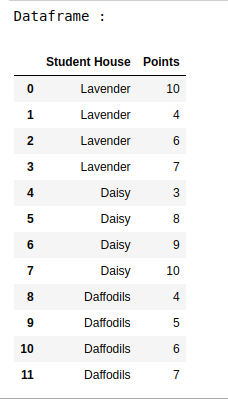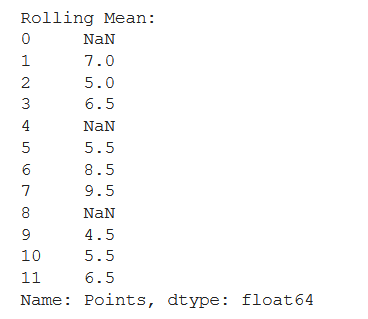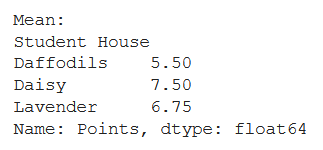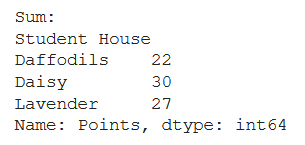Open in App
Not now

# How to apply functions in a Group in a Pandas DataFrame?

• Last Updated : 03 Jan, 2021

In this article, let’s see how to apply functions in a group in a Pandas Dataframe. Steps to be followed for performing this task are –

• Import the necessary libraries.
• Set up the data as a Pandas DataFrame.
• Use apply function to find different statistical measures like Rolling Mean, Average, Sum, Maximum, and Minimum. You can use the lambda function for this.

Below is the implementation-

Let’s create the dataframe.

## Python3

 `#import libraries``import` `pandas as pd`` ` `# set up the data``data_dict ``=` `{``"Student House"``: [``"Lavender"``, ``"Lavender"``, ``"Lavender"``,``                               ``"Lavender"``, ``"Daisy"``, ``"Daisy"``, ``                               ``"Daisy"``, ``"Daisy"``, ``"Daffodils"``, ``                               ``"Daffodils"``, ``"Daffodils"``, ``"Daffodils"``],``              ` `             ``"Points"``: [``10``, ``4``, ``6``, ``7``, ``3``, ``8``, ``9``, ``10``, ``4``, ``5``, ``6``, ``7``]}`` ` `data_df ``=` `pd.DataFrame(data_dict)``print``(``"Dataframe : "``)``data_df`

Output:Example 1:

## Python3

 `# finding rolling mean``rolling_mean ``=` `data_df.groupby(``"Student House"``)[``"Points"``].``apply``(``    ``lambda` `x: x.rolling(center``=``False``, window``=``2``).mean())`` ` `print``(``"Rolling Mean:"``)``print``(rolling_mean)`

Output:Example 2:

## Python3

 `# finding mean``mean ``=` `data_df.groupby(``"Student House"``)[``"Points"``].``apply``(``  ``lambda` `x: x.mean())`` ` `print``(``"Mean:"``)``print``(mean)`

Output:Example 3:

## Python3

 `# finding sum``sum` `=` `data_df.groupby(``"Student House"``)[``"Points"``].``apply``(``  ``lambda` `x: x.``sum``())`` ` `print``(``"Sum:"``)``print``(``sum``)`

Output:My Personal Notes arrow_drop_up